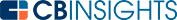# What is the Covariance Formula?The covariance formula is a numerical representation of the relationship between two random variables. It’s regularly used in investing, particularly in portfolio theory and diversification.

Covariance shows whether two variables tend to break in the same or opposite directions. When one variable moves in a particular direction, covariance shows the tendency of the second variable to move in the same or opposite direction. Taken alone, the figure does not show dependence or causality between variables, but it’s still a beneficial tool in portfolio management.

As it can identify assets with inverse price movements, covariance can be used to diversify a portfolio and minimize unsystematic risk.

## What is the covariance formula?

The covariance formula is an equation used to determine the directional relationship of two variables. It gives a figure between -1 and 1. Negative values show that the variables tend to move in opposite directions, while positive values indicate that they move in the same direction. The covariance formula uses individual points, so outliers can have a significant overall impact on the measurement.

In trading, covariance is used as a quick method of determining whether an asset can be used to reduce a portfolio’s overall risk position. You can combine it with the correlation coefficient for more effective results.

There are two covariance formulas used to calculate covariance. While similar, one is used for population samples while the other is used for variance samples.

To calculate the covariance between two random population covariables, you can use the following population covariance formula:To calculate sample covariance, you can use the following sample covariance formula:Where:

xi = Data variable of x

yi = Data variable of y

x = Mean of x

y = Mean of y

n = Number of data variables

## What covariance shows us

Covariance is represented as a value between -1 and 1. It shows the following relationships:

• Positive covariance — A positive covariance, greater than 0 and as high as 1, means that the two values tend to move in the same direction.
• Negative covariance — A negative covariance, between 0 and -1, means that the variables will move in opposite directions. For example, stock A’s price falls when stock B’s price rises.
• Zero covariance — Zero covariance means that there is no discernible link and the two values should be treated as independent.

While covariance shows the prevalence of a relationship between variables, it does not show dependency or causality. Consequently, it can’t answer the question of whether the movement of one price is caused or affected by the movement of the other price. However, there is a way to determine this: combine the covariance with the correlation coefficient.

## Uses of the covariance formula

Covariance is most often used in conjunction with correlation. While covariance only shows the direction of variation, correlation shows the strength of the relationship. In investment, covariance is most often used in portfolio management to help eliminate unsystematic risk.

For example, an investor can determine the covariance of a proposed asset compared to their overall portfolio. A negative covariance will show that the proposed asset is likely to perform well when the portfolio is suffering a downturn. In short, covariance offers a quick means of reducing portfolio risk.

It’s important to keep in mind that covariance is not a measure of the strength of the relationship between variables (unless combined with the correlation coefficient).

The covariance formula can be useful for investors looking to reduce unsystematic risk. In order to make an accurate calculation, remember to use the appropriate formula for your situation — either the population covariance formula or the sample covariance formula.

You may also like# www.cbinsights.com